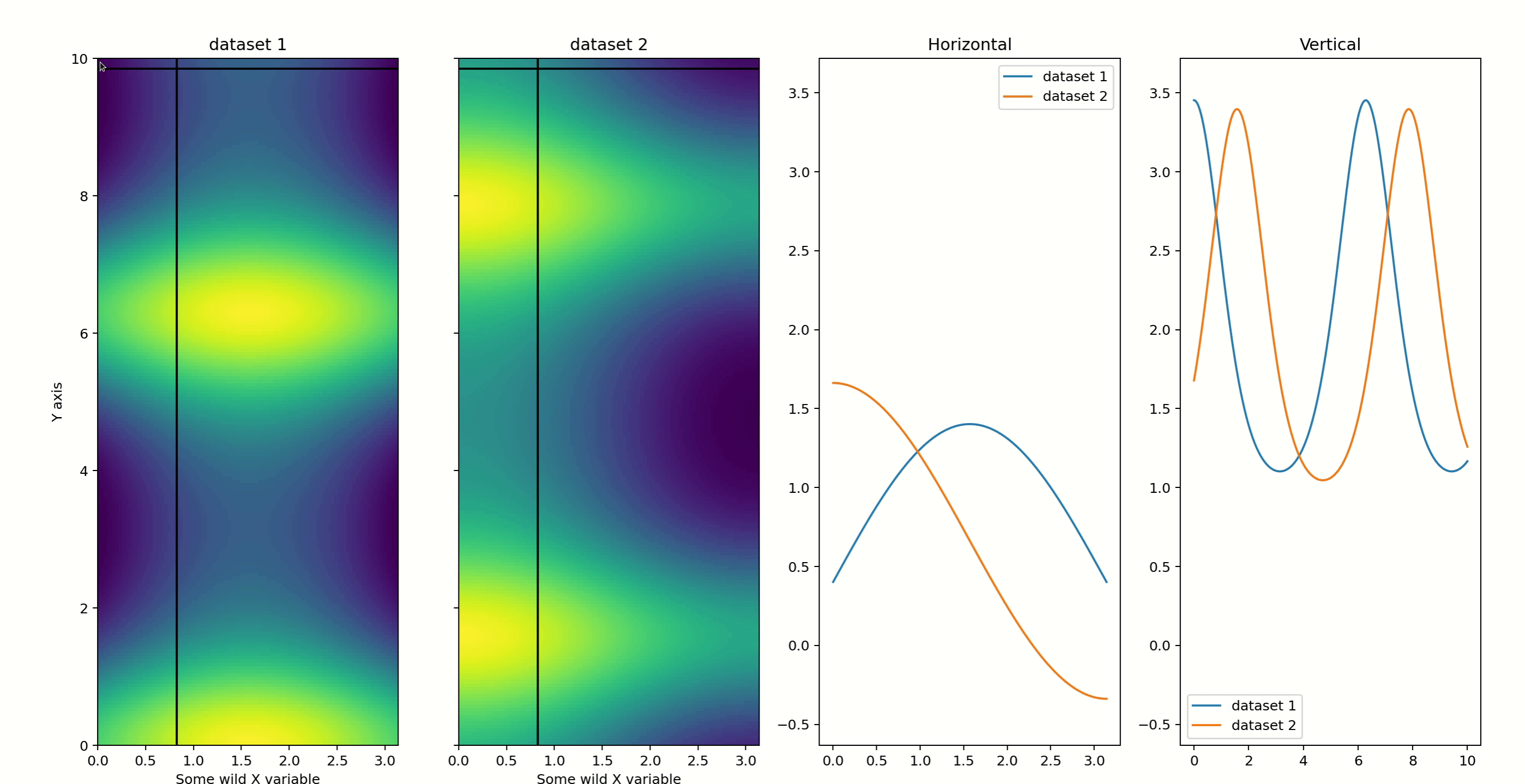# Compare Slices of 2D Arrays¶

Note

Unfortunately the interactive plots do not work on a website because there is no Python kernel running. All of the interactive outputs have therefore been replaced by gifs of what you should expect.

The `heatmap_slicer()` function allow you to compare horizontal and/or vertical slices of an arbitrary number of 2D arrays using just your mouse.

```x = np.linspace(0, np.pi, 100)
y = np.linspace(0, 10, 200)
X,Y = np.meshgrid(x, y)
data1 = np.sin(X) + np.exp(np.cos(Y))
data2 = np.cos(X) + np.exp(np.sin(Y))
fig, axes = heatmap_slicer(x, y, (data1,data2),slices='both',
heatmap_names=('dataset 1','dataset 2'),
labels=('Some wild X variable','Y axis'),
interaction_type='move')
```## Options¶

The `interaction_type` argument controls how the plot updates. You can either use `'move'` in which case all mouse movements will be tracked, or you can use `'click'` and the plot will only update when you click on one of the arrays.

The `slices` argument controls which slices to compare. It accepts values of `'vertical'`, `'horizontal'`, and `'both'`.

## Potential improvements¶

Do you wish the `heatmap_slicer` was better or worked with arbitrary angles? Then you should consider helping out on one of the open issues for improving it!